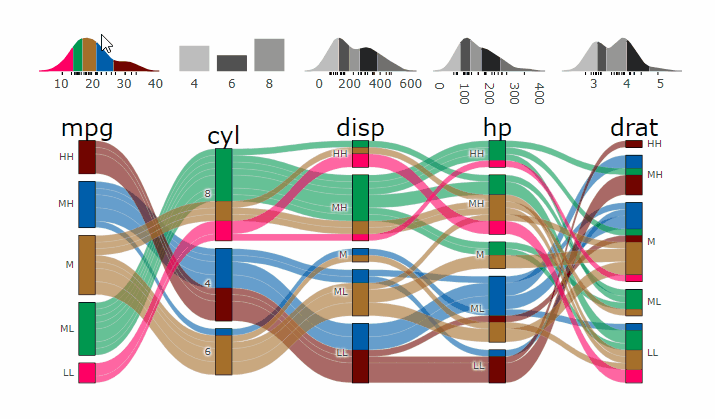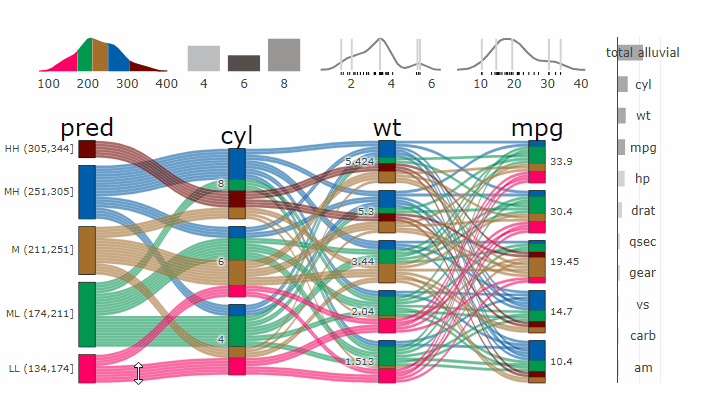# parcats###### Create ‘plotly.js’ Parallel Categories Diagrams Using this Htmlwidget and ‘easyalluvial’

Complex graphical representations of data are best explored using interactive elements. ‘parcats’ adds interactive graphing capabilities to the ‘easyalluvial’ package. The ‘plotly.js’ parallel categories diagrams offer a good framework for creating interactive flow graphs that allow manual drag and drop sorting of dimensions and categories, highlighting single flows and displaying mouse over information. The ‘plotly.js’ dependency is quite heavy and therefore is outsourced into a separate package.

## Installation

### CRAN

``install.packages('parcats')``

### Development Version

``````# install.packages("devtools")
devtools::install_github("erblast/parcats")``````

## easyalluvial

`parcats` requires an alluvial plot created with `easyalluvial` to create an interactive parrallel categories diagram.

## Examples

``````suppressPackageStartupMessages(require(tidyverse))
suppressPackageStartupMessages(require(easyalluvial))
suppressPackageStartupMessages(require(parcats))``````

### Shiny Demo

The shiny demo allows you to interactively explore the parameters of `alluvial_wide()` and `parcats()`

``parcats_demo()``

### Live Widget

The Htmlwidgets cannot be embedded in the `README.md` file. Check out the Live Widget here.

### Parcats from alluvial from data in wide format

``````p <- alluvial_wide(mtcars2, max_variables = 5)

parcats(p, marginal_histograms = TRUE, data_input = mtcars2)``````### Parcats from model response alluvial

Machine Learning models operate in a multidimensional space and their response is hard to visualise. Model response and partial dependency plots attempt to visualise ML models in a two dimensional space. Using alluvial plots or parrallel categories diagrams we can increase the number of dimensions.

Here we see the response of a random forest model if we vary the three variables with the highest importance while keeping all other features at their median/mode value.

``````df <- select(mtcars2, -ids )
m <- randomForest::randomForest( disp ~ ., df)
imp <- m\$importance
dspace <- get_data_space(df, imp, degree = 3)
pred <- predict(m, newdata = dspace)
p <- alluvial_model_response(pred, dspace, imp, degree = 3)

parcats(p, marginal_histograms = TRUE, imp = TRUE, data_input = df)``````